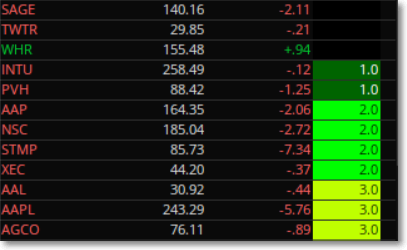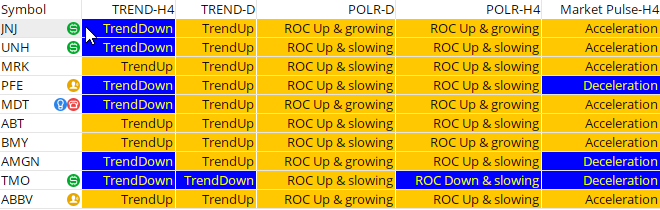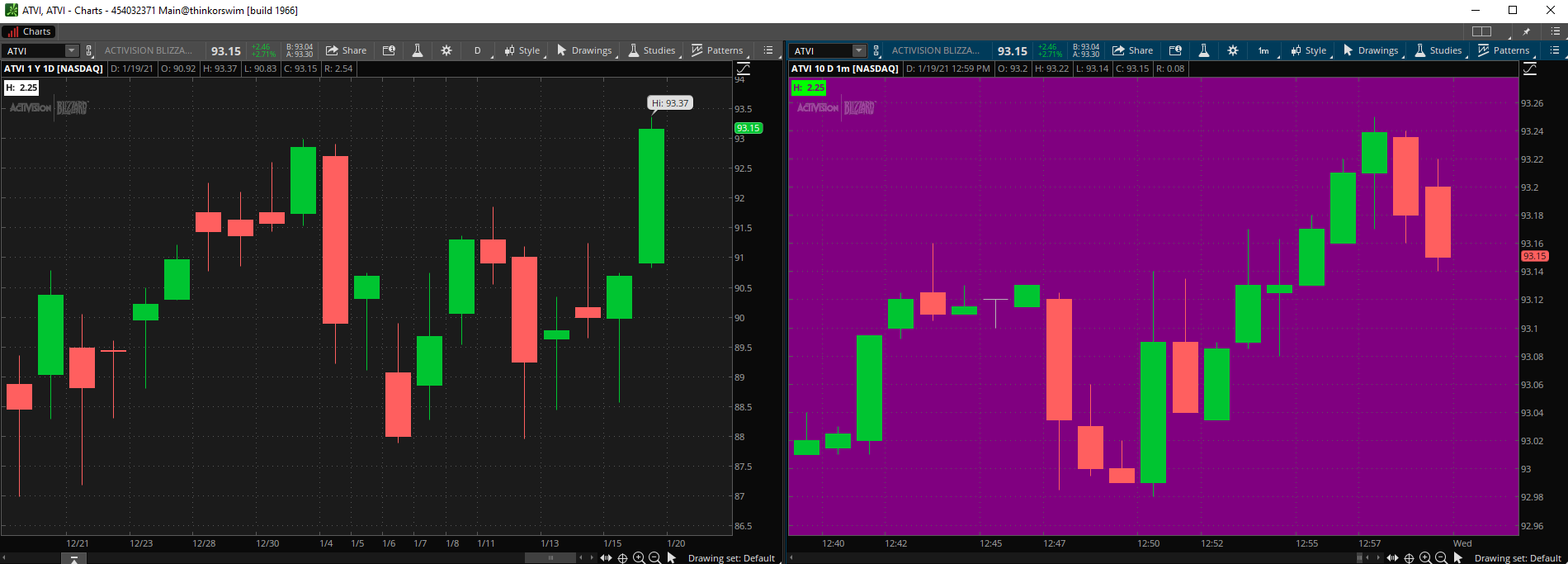# ThinkorSwim AssignBackgroundColor() Code Issue

##### New member
VIP
I have a Custom WatchList Column linked to a Scan that displays a 1.0 if true and a different color. I want to improve the Column with a Checklist or Grading of the Scan results.

I want to add code that displays the Sum of additional Conditions in the Column

Code:
``````If “Condition2" is true then count 1+1=2.0, else 1+0=1.0;
If “Condition3" is true then count +1, else +0;``````

So the column will display a 1.0 or 2.0 or 3.0. Can you help with the count code please?

Ruby:
``````def ma8 = Average(close, 8);
def ma13 = Average(close, 13);
def ma21 = Average(close, 21);

def cond1 = close > ma8;
def cond2 = close > ma13;
def cond3 = close > ma21;

plot total = cond1 + cond2 + cond3;
total.AssignValueColor(if total == 1 then Color.WHITE else Color.BLACK);
AssignBackgroundColor(if total == 3 then Color.LIME else if total == 2 then Color.GREEN else if total == 1 then Color.DARK_GREEN else Color.BLACK);``````plot total = cond1 + cond2 + cond3;
A simple solution. With a color-coded option. Thank you.

•markos
I want to assign value colors on my watch list. I am looking at the 50 day volume percent change and 50 day price percent change and assigning a color based on the value being a positive or negative percent. Here is the script that I have so far. The problem seems to be on the last line of script. I need to add a word or something in front of the AssignValueColor. Here is the script.

Code:
``````input length = 50;
def vol = volume;
def volAvg = Average( volume, length );
def volPctChg = 100 * ( vol - volAvg ) / volAvg;
plot VolumePercentChange = Round( volPctChg, 0 );

input lookback = 50;
def price = close;
def priceAvg = Average( price, length );
def pricePctChg = 100 * ( price - priceAvg ) / priceAvg;
plot pricePercentChange = Round( pricePctChg, 0 );

AssignVallueColor (if pricePctChg >=1 and volPctChg <= 1 then color.Yellow else color.White);``````

Here is an example... I didn't verify your logic...

`pricePercentChange.AssignValueColor(if pricePctChg >=1 and volPctChg <= 1 then color.Yellow else color.White);`

The line of script you wrote worked. However, it now says "Exactly one plot expected"
Any suggestions?

@Rider, change one of your "plot" to "def"

Post your current code and we'll take a look...

Edited to add: The following code works for me...

Code:
``````input length = 50;
def vol = volume;
def volAvg = Average( volume, length );
def volPctChg = 100 * ( vol - volAvg ) / volAvg;
plot VolumePercentChange = Round( volPctChg, 0 );

input lookback = 50;
def price = close;
def priceAvg = Average( price, length );
def pricePctChg = 100 * ( price - priceAvg ) / priceAvg;
plot pricePercentChange = Round( pricePctChg, 0 );

pricePercentChange.AssignValueColor(if pricePctChg >=1 and volPctChg <= 1 then color.Yellow else color.White);``````

Last edited:
@rad14733 He is doing a Watchlist. Watchlists by definition can only plot one item. The VolumePercentChange or pricePercentChange.
Seeing as he is assigning the color value to pricePercentChange, I am assuming that is the number he wants to see. Therefore he needs to change the plot before VolumePercentChange to def

Works! I changed one of the plots to a def. as suggested. Thank you.

@rad14733 He is doing a Watchlist. Watchlists by definition can only plot one item. The VolumePercentChange or pricePercentChange.
Seeing as he is assigning the color value to pricePercentChange, I am assuming that is the number he wants to see. Therefore he needs to change the plot before VolumePercentChange to def
True... Forgot he wanted this for a Watchlist rather than on a chart, which is where I tested...

I have a custom quote script that one of the addlabel is not getting assigned the correct background color. The condition for "ROCup_slowing" label is not getting the blue color background. Not sure why this is so. Any assistance would be appreciated. Thanks

ROC Up & slowing should be blue and ROC Down & slowing should be orange.Code:
``````def AO = Average(hl2, 5) - Average(hl2, 34);
def Zero = 0;

plot signalline = Average(AO, 5);

def ROCup_Accelerating = if AO > zero then 1 else 0;
def ROCup_Slowing = if AO > zero and AO < signalline then 1 else 0;
def ROCdown_Accelerating = if AO < zero then 1 else 0;
def ROCdown_Slowing = if AO < zero and AO > signalline then 1 else 0;

AddLabel(ROCup_Accelerating, "ROC Up & growing", color.black);
AddLabel(ROCup_Slowing, "ROC Up & slowing", color.black);
AddLabel(ROCdown_Accelerating, "ROC Down & growing", color.yellow);
AddLabel(ROCdown_Slowing, "ROC Down & slowing", color.yellow);

AssignBackgroundColor(if ROCup_accelerating then color.orange else if ROCup_Slowing then color.blue else if ROCdown_Accelerating then color.blue else color.orange);``````

@liminal-rider You need to change your definitions just a bit.

Code:
``````def ROCup_Accelerating = if AO > Zero and AO > signalline then 1 else 0;
def ROCup_Slowing = if AO > Zero and AO < signalline then 1 else 0;
def ROCdown_Accelerating = if AO < Zero and AO < signalline then 1 else 0;
def ROCdown_Slowing = if AO < Zero and AO > signalline then 1 else 0;``````

Brilliant OBW...amazing how some times the change is right in front of our eyes...Thank you so much for your fresh viewpoint.

Hi I’m new to creating script and hoping someone can tell me what’s wrong with this code. Its purpose is twofold:
• Add a label to show the difference between yesterday’s high + \$0.15 and the current last price
• Change the color of the label and of the chart if that difference is between -\$0.50 and \$0.20
It seems to work fine on a daily chart, but not the 1 minute chart and that’s what I need it for. The issue is that the label and chart change color on the 1 min even though the condition hasn’t been met.

Any help is appreciated.

Code:
``````def Yhigh = high(period = aggregationPeriod.Day) + .15;
input priceType = PriceType.LAST;
def LastPrice = close(priceType = priceType);
def difference = LastPrice - Yhigh;
def InRange = difference between -.50 and .20;
AddLabel(yes, Concat("H:  ", difference), ((if InRange then Color.GREEN else Color.WHITE)));
assignbackgroundColor(if InRange then Color.PLUM else Color.Current);``````

The chart has to be able to see yesterdays high, if the chart cant see yesterday's price then neither will the script.

Thanks but I'm not sure that's the solution, or maybe I'm missing something. For example, if you put a daily chart and a 1 min chart next to each other of the same stock, I can get the exact "difference" value by extending the timeframe of the min chart more than a day back and eliminating the extended hours trading. But while the "difference" value is the same, the min chart will be colored whereas the daily will not, and I'm not sure why that is.

@OC_Trader The AssignBackgroundColor() is for changing the Chart Background, not a Label... Use AssignValueColor() instead for Charts... However, AssignBackgroundColor() will work for Watchlist Columns...

But if that's true why does it work fine for the daily chart? It seems only when I use a lower timeframe I have issues.Code:
``def LastPrice = close(priceType = priceType);``

to

Code:
``def LastPrice = close;``

### Not the exact question you're looking for?

87k+ Posts
343 Online## The Market Trading Game Changer

Join 2,500+ subscribers inside the useThinkScript VIP Membership Club
• Exclusive indicators
• Proven strategies & setups
• Private Discord community
• Exclusive members-only content
• 1 full year of unlimited support

What is useThinkScript?

useThinkScript is the #1 community of stock market investors using indicators and other tools to power their trading strategies. Traders of all skill levels use our forums to learn about scripting and indicators, help each other, and discover new ways to gain an edge in the markets.

How do I get started?

We get it. Our forum can be intimidating, if not overwhelming. With thousands of topics, tens of thousands of posts, our community has created an incredibly deep knowledge base for stock traders. No one can ever exhaust every resource provided on our site.

If you are new, or just looking for guidance, here are some helpful links to get you started.

What are the benefits of VIP Membership?